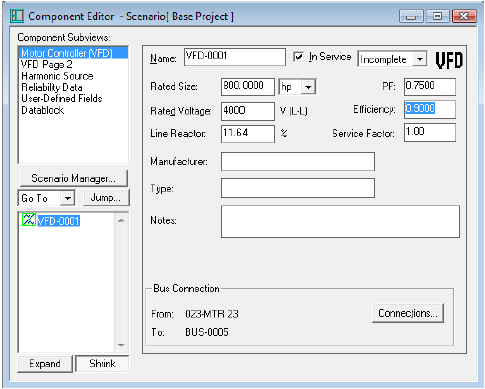How do I enter manufacturer provided AC reactor value (in mh) for VFD components?

The Line Reactor % refers to the Voltage Drop %.

For Example:

A 6.2mh AC reactor in the following Motor: 800hp, 4000V & 0.75pf would have 115amps (FLA).

A 6.2mh AC reactor is 2 x (pi) x 60hz x 6.2 mh / 1000 = 2.337Ohms

The Voltage Drop is 115A x (1.732) x 2.337ohms = 465.48Volts

The data entered into line reactor % should then be 465.48V / 4000V x 100 = 11.637%